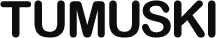Home Page

# Clumpy Reference

## Clumpy Has Moved

It’s here now: https://thomasperi.github.io/clumpyjs/reference

This page documents Clumpy, method by method. The examples are out of context and show only the invocation of the method. See the usage guide for more information on how to use these methods together.

These examples also suppose that you have a function called `output` that inserts text into some element on the page.

## Constructor

`new Clumpy(options)`

Creates a new `Clumpy` instance.

### Parameters

(optional) `options` An object with options as its properties. A function to call between clumps. Default is a function that does nothing. The number of milliseconds to rest between clumps. Default is 0. The maximum number of milliseconds each clump is allowed to run. Default is 100.

### Example

``````// Defaults:
new Clumpy()

// With Options Specified:
new Clumpy({
between: function () {
output('Progress is being made, I promise.');
},
// devotes equal time to processing and resting
delay: 50,
duration: 50
})``````

## Instance Methods

`break_loop(label)`

Breaks the closest loop with the specified label, or the current loop if no label is given.

### Parameters

(optional) `label` The label of the loop to break.
`continue_loop(label)`

Continues the closest loop with the specified label, or the current loop if no label is given.

### Parameters

(optional) `label` The label of the loop to continue.
`do_while_loop(statements, test)`

Models a `do...while` loop.

### Parameters

`statements` A function that performs one iteration of the loop’s body. A function that returns the result of the condition for the loop.

### Example

``````// Real Loop
do {
output(i);
i += 1;
} while (i < 10);

// Clumpy Equivalent
do_while_loop(
function () {
output(i);
i += 1;
},
function () { return i < 10; }
)``````
`for_in_loop(objectFn, statements)`

Models a `for...in` loop.

### Parameters

`objectFn` A function that returns the object over which to iterate. A function that performs one iteration of the loop’s body. It should accept an argument representing the key for the current loop iteration.

### Example

``````// Real Loop
for (key in object) {
output(key + ': ' + object[key]);
}

// Clumpy Equivalent
for_in_loop(
function () { return object; },
function (k) {
key = k;
output(key + ': ' + object[key]);
}
)``````

The scratch variable `k` in the example above is for mimicking the scope `key` has in the real loop. If you don’t need to access the variable from outside the `statements` function, you can use its argument directly:

``````for_in_loop(
function () { return object; },
function (key) {
output(key + ': ' + object[key]);
}
)``````
`for_loop(init, test, inc, statements)`

Models a `for` loop.

### Parameters

`init` A function that initializes the loop. A function that returns the result of the condition for the loop. A function that increments the loop. A function that performs one iteration of the loop’s body.

### Example

``````// Real Loop
for (i = 0; i < 10; i += 1) {
output(i);
}

// Clumpy Equivalent
for_loop(
function () { i = 0; },
function () { return i < 10; },
function () { i += 1; },
function () {
output(i);
}
)``````
`init()`

Stop and forget everything this Clumpy instance is doing, but don’t reset the options the user has set.

`interrupt()`

Enqueue an interrupt—an end to the clump that’s running when the interrupt is dequeued.

`label(name)`

Assigns a label to the next unit enqueued, if the unit is a loop. If the unit is not a loop, the label is discarded.

### Parameters

`name` The string to assign as the label.
`once(statements)`

Calls the supplied function a single time.

### Parameters

`statements` The function to invoke.
`pause()`

Halts execution until `resume()` is called.

Note: `pause()` and `resume()` are for external control over a Clumpy instance, and should generally not be used inside a loop. They do not enqueue their actions, but instead act at the time they are invoked.

`resume()`

Resumes execution after `pause()`.

See the note under `pause()`.

`set(options)`

Enqueue the setting of options for this Clumpy instance. Contrast this with `setNow` below, which sets the options at the time the method is called.

### Parameters

`options` An object in the same form as that which can be passed to the `Clumpy` constructor.
`setNow(options)`

Set options for this Clumpy instance NOW. Contrast this with `set` above, which doesn’t set the options immediately, but enqueues the act of setting the options.

### Parameters

`options` An object in the same form as that which can be passed to the `Clumpy` constructor.
`sleep(delay)`

Halts execution and resumes after the specified delay.

### Parameters

`delay` The number of milliseconds to halt execution.
`wait(statements)`

Halts execution until an asynchronous operation is complete.

### Parameters

`statements` The function to call. It should accept a function as an argument, and invoke that function as a callback when the task is complete.

### Example

``````wait(function (callback) {
jQuery.get('example.php', function (data) {
output('Got Data: ' + data);
callback();
});
})``````
`while_loop(test, statements)`

Models a `while` loop.

### Parameters

`test` A function that returns the result of the condition for the loop. A function that performs one iteration of the loop’s body.

### Example

``````// Real Loop
while (i < 10) {
output(i);
i += 1;
}

// Clumpy Equivalent
while_loop(
function () { return i < 10; },
function () {
output(i);
i += 1;
}
)``````
© Thomas Peri